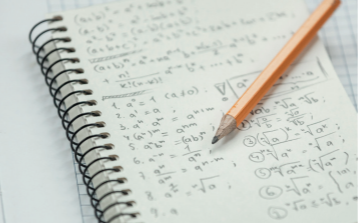# A-LEVEL Further Mathematics

A-LEVEL

The Further Mathematics course covers both A-Level Mathematics and A-Level Further Mathematics. It extends the understanding gained in A-Level Mathematics to higher levels, as well as exploring new topics, such as imaginary numbers. It is advantageous to students considering studying Mathematics at university. Also, students wishing to study Physics or Engineering will benefit from meeting the Mathematics they might need at this stage of their education. Students who select A-Level Further Mathematics as one of their choices should not select A-Level Mathematics as well, as A-Level Mathematics is incorporated into the Further Mathematics course. Please note that A-Level Further Mathematics counts as a double subject (two A-Levels).Subject requirements:

Minimum of GCSE 8 in Maths

Teacher to contact - Mrs S Mortimer-Billings
Area Coordinator of Maths - Mr J Dunning
Maths Curriculum Assistant - Mrs S Gardner

Course Content

Year 12

The course will introduce topics that are not covered in the regular Mathematics A-Level, with an emphasis on logical deduction, constructing mathematical arguments and proof. New content here will include complex numbers and matrices, as well as proof by induction. The course will also introduce new statistical processes.

Year 13

In Year 13, some ideas met in Year 12 are extended. This includes further complex numbers and matrices. New integration techniques are also taught here. In addition, the modelling techniques used in the statistics and mechanics section of the Maths A-Level are refined and extended, and new ideas fundamental to undergraduate Mathematics are explored.

Assessment Criteria

All content is assessed at the end of the course in four written examinations, in addition to the three examinations for A Level Maths. There is no coursework element.

Exam Board: MEI B (Examined through OCR)

• Economics and Finance
• Computing
• Medicine
• Statistical Research
• Engineering

This subject goes well with:

• Physics
• Biology
• Computer Science

Click below to learn about the course from Further Maths student, Lizzie